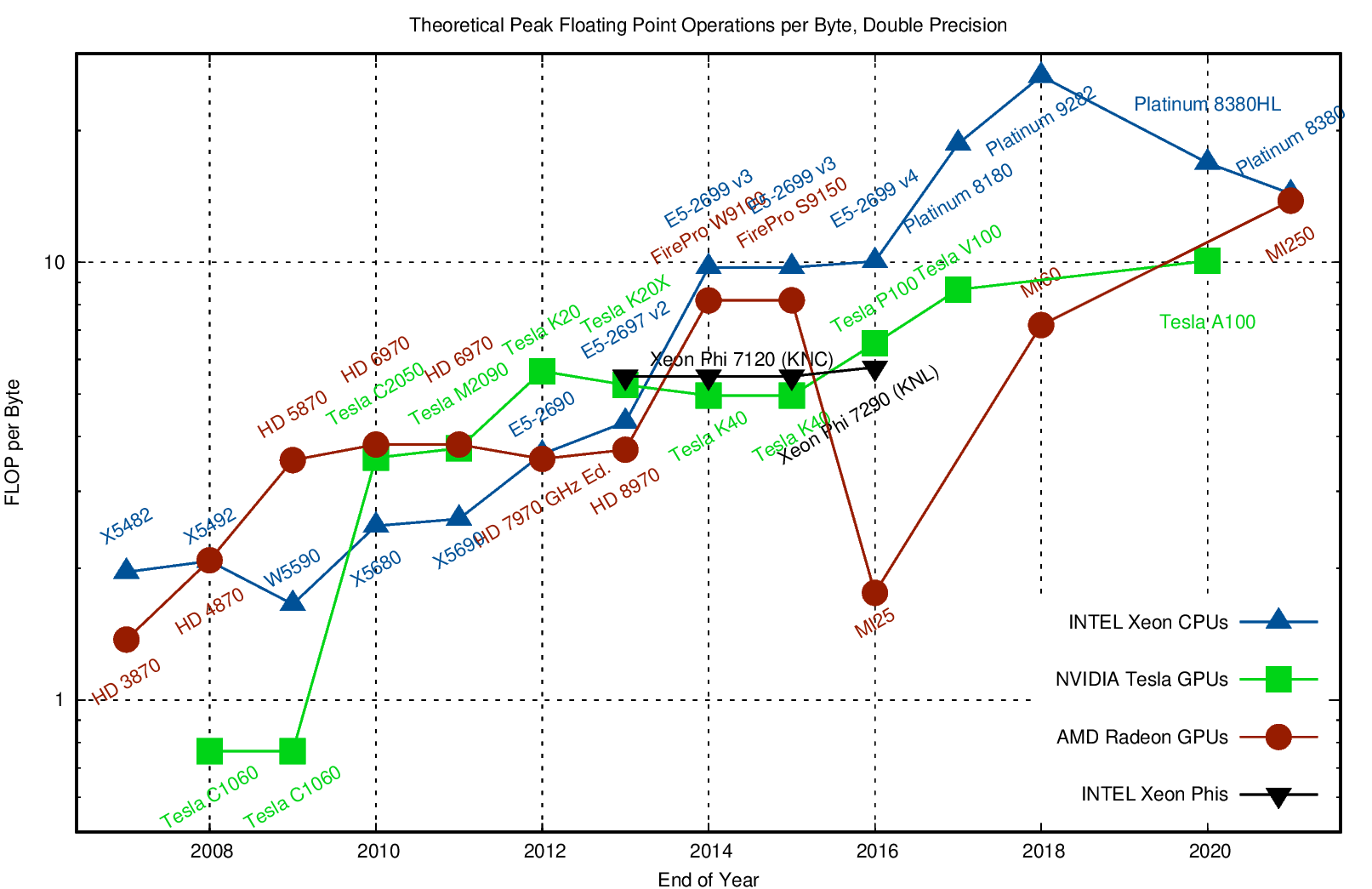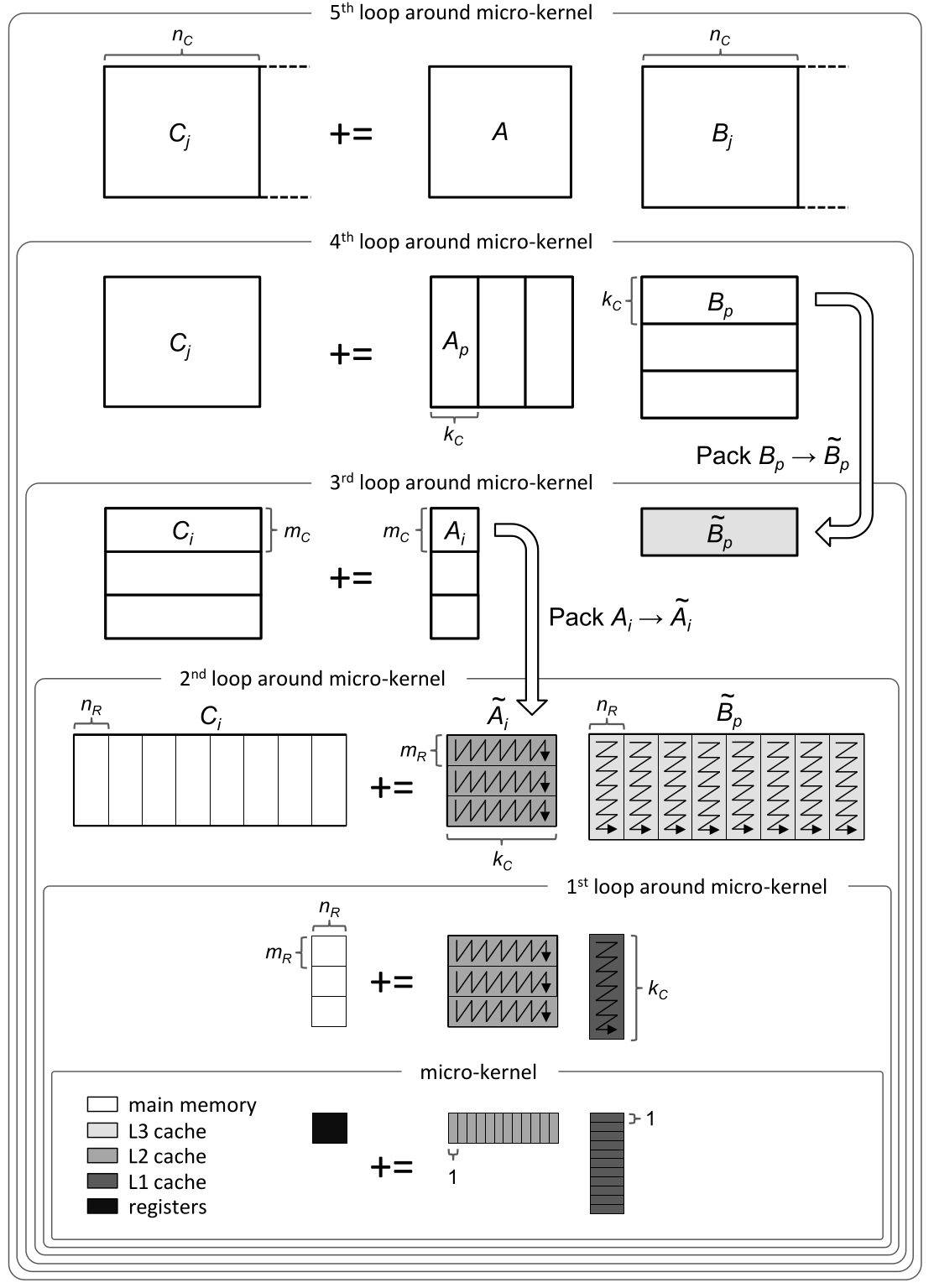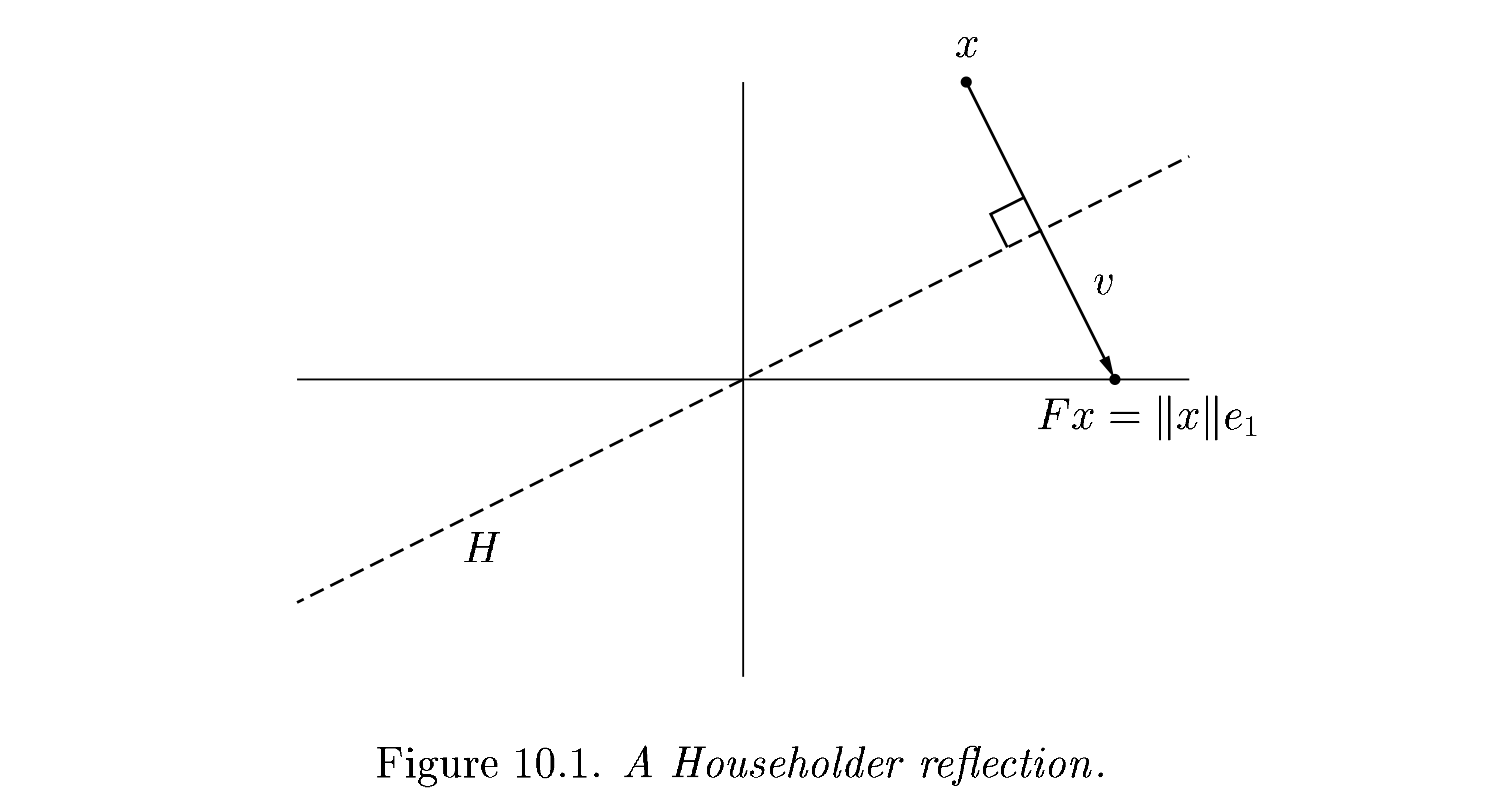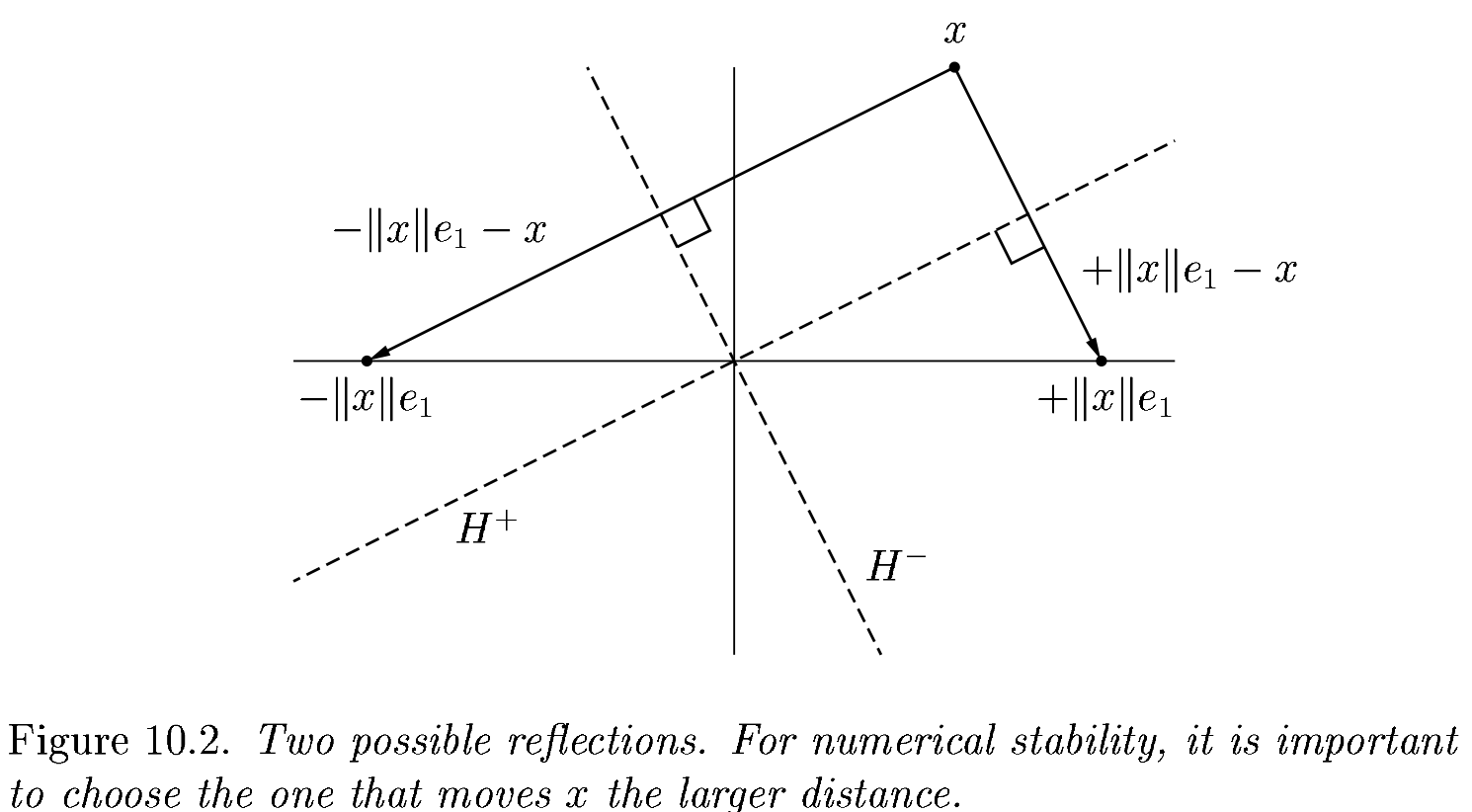# 2022-02-18 Householder QR¶

## Last time¶

• Stability and ill conditioning

• Intro to performance modeling

• Classical vs Modified Gram-Schmidt

## Today¶

• Performance strategies

• Right vs left-looking algorithms

• Elementary reflectors

• Householder QR

using LinearAlgebra
using Plots
using Polynomials
default(linewidth=4, legendfontsize=12)

function vander(x, k=nothing)
if isnothing(k)
k = length(x)
end
m = length(x)
V = ones(m, k)
for j in 2:k
V[:, j] = V[:, j-1] .* x
end
V
end

vander (generic function with 2 methods)


# A Gram-Schmidt with more parallelism¶

(12)\begin{align} (I - q_2 q_2^T) (I - q_1 q_1^T) v &= (I - q_1 q_1^T - q_2 q_2^T + q_2 q_2^T q_1 q_1^T) v \\ &= \Bigg( I - \Big[ q_1 \Big| q_2 \Big] \begin{bmatrix} q_1^T \\ q_2^T \end{bmatrix} \Bigg) v \end{align}
function gram_schmidt_classical(A)
m, n = size(A)
Q = zeros(m, n)
R = zeros(n, n)
for j in 1:n
v = A[:,j]
R[1:j-1,j] = Q[:,1:j-1]' * v
v -= Q[:,1:j-1] * R[1:j-1,j]
R[j,j] = norm(v)
Q[:,j] = v / R[j,j]
end
Q, R
end

gram_schmidt_classical (generic function with 1 method)

m = 20
x = LinRange(-1, 1, m)
A = vander(x, m)
Q, R = gram_schmidt_classical(A)
@show norm(Q' * Q - I)
@show norm(Q * R - A)

norm(Q' * Q - I) = 1.4985231287367549
norm(Q * R - A) = 7.350692433565389e-16

7.350692433565389e-16


# Why does order of operations matter?¶

(13)\begin{align} (I - q_2 q_2^T) (I - q_1 q_1^T) v &= (I - q_1 q_1^T - q_2 q_2^T + q_2 q_2^T q_1 q_1^T) v \\ &= \Bigg( I - \Big[ q_1 \Big| q_2 \Big] \begin{bmatrix} q_1^T \\ q_2^T \end{bmatrix} \Bigg) v \end{align}

is not exact in finite arithmetic.

# We can look at the size of what’s left over¶

We project out the components of our vectors in the directions of each $$q_j$$.

x = LinRange(-1, 1, 20)
A = vander(x)
Q, R = gram_schmidt_classical(A)
scatter(diag(R), yscale=:log10)# The next vector is almost linearly dependent¶

x = LinRange(-1, 1, 20)
A = vander(x)
Q, _ = gram_schmidt_classical(A)
#Q, _ = qr(A)
v = A[:,end]
@show norm(v)
scatter(abs.(Q[:,1:end-1]' * v), yscale=:log10)

norm(v) = 1.4245900685395503# Cost of Gram-Schmidt?¶

• We’ll count flops (addition, multiplication, division*)

• Inner product $$\sum_{i=1}^m x_i y_i$$?

• Vector “axpy”: $$y_i = a x_i + y_i$$, $$i \in [1, 2, \dotsc, m]$$.

• Look at the inner loop:

for k in 1:j-1
r = Q[:,k]' * v
v -= Q[:,k] * r
R[k,j] = r
end# Counting flops is a bad model¶

• We load a single entry (8 bytes) and do 2 flops (add + multiply). That’s an arithmetic intensity of 0.25 flops/byte.

• Current hardware can do about 10 flops per byte, so our best algorithms will run at about 2% efficiency.

• Need to focus on memory bandwidth, not flops.

## Dense matrix-matrix mulitply¶# Inherent data dependencies¶

$\begin{split} \Bigg[ q_1 \Bigg| q_2 \Bigg| q_3 \Bigg| q_4 \Bigg| q_5 \Bigg] \begin{bmatrix} r_{11} & r_{12} & r_{13} & r_{14} & r_{15} \\ & r_{22} & r_{23} & r_{24} & r_{25} \\ & & r_{33} & r_{34} & r_{35} \\ &&& r_{44} & r_{45} \\ &&& & r_{55} \end{bmatrix} \end{split}$

# Right-looking modified Gram-Schmidt¶

function gram_schmidt_modified(A)
m, n = size(A)
Q = copy(A)
R = zeros(n, n)
for j in 1:n
R[j,j] = norm(Q[:,j])
Q[:,j] /= R[j,j]
R[j,j+1:end] = Q[:,j]'*Q[:,j+1:end]
Q[:,j+1:end] -= Q[:,j]*R[j,j+1:end]'
end
Q, R
end

gram_schmidt_modified (generic function with 1 method)

m = 20
x = LinRange(-1, 1, m)
A = vander(x, m)
Q, R = gram_schmidt_modified(A)
@show norm(Q' * Q - I)
@show norm(Q * R - A)

norm(Q' * Q - I) = 8.486718528276085e-9
norm(Q * R - A) = 8.709998074379606e-16

8.709998074379606e-16


# Classical versus modified?¶

• Classical

• Really unstable, orthogonality error of size $$1 \gg \epsilon_{\text{machine}}$$

• Don’t need to know all the vectors in advance

• Modified

• Needs to be right-looking for efficiency

• Less unstable, but orthogonality error $$10^{-9} \gg \epsilon_{\text{machine}}$$

m = 20
x = LinRange(-1, 1, m)
A = vander(x, m)
Q, R = qr(A)
@show norm(Q' * Q - I)

norm(Q' * Q - I) = 3.1195004131362406e-15

3.1195004131362406e-15


# Householder QR¶

Gram-Schmidt constructed a triangular matrix $$R$$ to orthogonalize $$A$$ into $$Q$$. Each step was a projector, which is a rank-deficient operation. Householder uses orthogonal transformations (reflectors) to triangularize.

$\underbrace{Q_{n} \dotsb Q_1}_{Q^T} A = R$

The structure of the algorithm is

$\begin{split} \underbrace{\begin{bmatrix} * & * & * \\ * & * & * \\ * & * & * \\ * & * & * \\ * & * & * \\ \end{bmatrix}}_{A} \to \underbrace{\begin{bmatrix} * & * & * \\ 0 & * & * \\ 0 & * & * \\ 0 & * & * \\ 0 & * & * \\ \end{bmatrix}}_{Q_1 A} \to \underbrace{\begin{bmatrix} * & * & * \\ 0 & * & * \\ 0 & 0 & * \\ 0 & 0 & * \\ 0 & 0 & * \\ \end{bmatrix}}_{Q_2 Q_1 A} \to \underbrace{\begin{bmatrix} * & * & * \\ 0 & * & * \\ 0 & 0 & * \\ 0 & 0 & 0 \\ 0 & 0 & 0 \\ \end{bmatrix}}_{Q_3 Q_2 Q_1 A} \end{split}$

# Constructing the $$Q_j$$¶

$\underbrace{Q_{n} \dotsb Q_1}_{Q^T} A = R$

Each of our $$Q_j$$ will have the form

$\begin{split}Q_j = \begin{bmatrix} I_i & 0 \\ 0 & F \end{bmatrix}\end{split}$
where $$F$$ is a “reflection” that achieves
$\begin{split} F x = \begin{bmatrix} \lVert x \rVert \\ 0 \\ 0 \\ \vdots \end{bmatrix} \end{split}$
where $$x$$ is the column of $$R$$ from the diagonal down. This transformation is a reflection across a plane with normal $$v = Fx - x = \lVert x \rVert e_1 - x$$.The reflection, as depicted above by Trefethen and Bau (1999) can be written $$F = I - 2 \frac{v v^T}{v^T v}$$.

# Adventures in reflection¶

A = rand(4, 4); A += A'
v = copy(A[:,1])
v -= norm(v)
v = normalize(v)
F = I - 2 * v * v'
B = F * A

4×4 Matrix{Float64}:
2.1741       1.25714    0.99767    1.18672
-6.55398e-16  0.118113   0.765884   1.28508
-2.96172e-16  1.05277   -0.0743418  0.52617
-8.46837e-16  0.985914   0.0179292  0.779973

v = copy(B[2:end, 2])
v -= norm(v); v = normalize(v)
F = I - 2 * v * v'
B[2:end, 2:end] = F * B[2:end, 2:end]
B

4×4 Matrix{Float64}:
2.1741       1.25714      0.99767   1.18672
-6.55398e-16  1.44717      0.020642  1.01903
-2.96172e-16  0.0          0.515979  0.736919
-8.46837e-16  1.11022e-16  0.570759  0.977337


# An algorithm¶

function qr_householder_naive(A)
m, n = size(A)
R = copy(A)
V = [] # list of reflectors
for j in 1:n
v = copy(R[j:end, j])
v -= norm(v)
v = normalize(v)
R[j:end,j:end] -= 2 * v * (v' * R[j:end,j:end])
push!(V, v)
end
V, R
end

qr_householder_naive (generic function with 1 method)

m = 4
x = LinRange(-1, 1, m)
A = vander(x, m)
V, R = qr_householder_naive(A)
_, R_ = qr(A)
R_

4×4 Matrix{Float64}:
-2.0  0.0      -1.11111      0.0
0.0  1.49071   1.38778e-17  1.3582
0.0  0.0       0.888889     9.71445e-17
0.0  0.0       0.0          0.397523


# How to interpret $$V$$ as $$Q$$?¶

function reflectors_mult(V, x)
y = copy(x)
for v in reverse(V)
n = length(v) - 1
y[end-n:end] -= 2 * v * (v' * y[end-n:end])
end
y
end

function reflectors_to_dense(V)
m = length(V)
Q = diagm(ones(m))
for j in 1:m
Q[:,j] = reflectors_mult(V, Q[:,j])
end
Q
end

reflectors_to_dense (generic function with 1 method)

m = 20
x = LinRange(-1, 1, m)
A = vander(x, m)
V, R = qr_householder_naive(A)
Q = reflectors_to_dense(V)
@show norm(Q' * Q - I)
@show norm(Q * R - A);

norm(Q' * Q - I) = 3.7994490775439526e-15
norm(Q * R - A) = 7.562760794606217e-15


# Great, but we can still break it¶

A = [1 0; 0 1.]
V, R = qr_householder_naive(A)

(Any[[NaN, NaN], [NaN]], [NaN NaN; NaN NaN])


We had the lines

    v = copy(R[j:end, j])
v -= norm(v)
v = normalize(v)


What happens when R is already upper triangular?# An improved algorithm¶

function qr_householder(A)
m, n = size(A)
R = copy(A)
V = [] # list of reflectors
for j in 1:n
v = copy(R[j:end, j])
v += sign(v) * norm(v) # <---
v = normalize(v)
R[j:end,j:end] -= 2 * v * v' * R[j:end,j:end]
push!(V, v)
end
V, R
end

qr_householder (generic function with 1 method)

A = [2 -1; -1 2] * 1e-10
V, R = qr_householder(A)
tau = [2*v^2 for v in V]
@show tau
V1 = [v ./ v for v in V]
@show V1
R

tau = [1.894427190999916, 2.0]
V1 = [[1.0, -0.2360679774997897], [1.0]]

2×2 Matrix{Float64}:
-2.23607e-10   1.78885e-10
-1.29247e-26  -1.34164e-10


# Householder is backward stable¶

m = 40
x = LinRange(-1, 1, m)
A = vander(x, m)
V, R = qr_householder(A)
Q = reflectors_to_dense(V)
@show norm(Q' * Q - I)
@show norm(Q * R - A);

norm(Q' * Q - I) = 5.949301496893686e-15
norm(Q * R - A) = 1.2090264267288813e-14

A = [1 0; 0 1.]
V, R = qr_householder(A)
qr(A)

LinearAlgebra.QRCompactWY{Float64, Matrix{Float64}}
Q factor:
2×2 LinearAlgebra.QRCompactWYQ{Float64, Matrix{Float64}}:
1.0  0.0
0.0  1.0
R factor:
2×2 Matrix{Float64}:
1.0  0.0
0.0  1.0


# Orthogonality is preserved¶

x = LinRange(-1, 1, 20)
A = vander(x)
Q, _ = gram_schmidt_classical(A)
v = A[:,end]
@show norm(v)
scatter(abs.(Q[:,1:end-1]' * v), yscale=:log10, title="Classical Gram-Schmidt")

norm(v) = 1.4245900685395503Q = reflectors_to_dense(qr_householder(A))
scatter(abs.(Q[:,1:end-1]' * v), yscale=:log10, title="Householder QR")